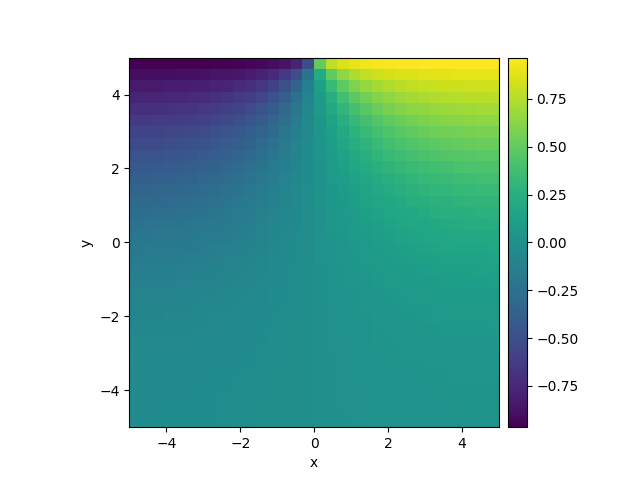# 2.13. Heterogeneous boundary conditions

This example implements a spatially coupled SIR model with the following dynamics for the density of susceptible, infected, and recovered individuals:

$\begin{split}\partial_t s &= D \nabla^2 s - \beta is \\ \partial_t i &= D \nabla^2 i + \beta is - \gamma i \\ \partial_t r &= D \nabla^2 r + \gamma i\end{split}$

Here, $$D$$ is the diffusivity, $$\beta$$ the infection rate, and $$\gamma$$ the recovery rate.0%|          | 0/10.0 [00:00<?, ?it/s]
Initializing:   0%|          | 0/10.0 [00:00<?, ?it/s]
0%|          | 0/10.0 [00:00<?, ?it/s]
2%|▏         | 0.24/10.0 [00:00<00:09,  1.02it/s]
7%|▋         | 0.71/10.0 [00:00<00:03,  2.55it/s]
30%|██▉       | 2.99/10.0 [00:00<00:01,  6.23it/s]
80%|████████  | 8.04/10.0 [00:00<00:00,  8.70it/s]
80%|████████  | 8.04/10.0 [00:01<00:00,  7.34it/s]
100%|██████████| 10.0/10.0 [00:01<00:00,  9.13it/s]
100%|██████████| 10.0/10.0 [00:01<00:00,  9.13it/s]


import numpy as np

from pde import CartesianGrid, DiffusionPDE, ScalarField

# define grid and an initial state
grid = CartesianGrid([[-5, 5], [-5, 5]], 32)
field = ScalarField(grid)

# define the boundary conditions, which here are calculated from a function
def bc_value(adjacent_value, dx, x, y, t):
"""return boundary value"""
return np.sign(x)

bc_x = "derivative"
bc_y = ["derivative", {"value_expression": bc_value}]

# define and solve a simple diffusion equation
eq = DiffusionPDE(bc=[bc_x, bc_y])
res = eq.solve(field, t_range=10, dt=0.01, backend="numpy")
res.plot()


Total running time of the script: (0 minutes 1.280 seconds)